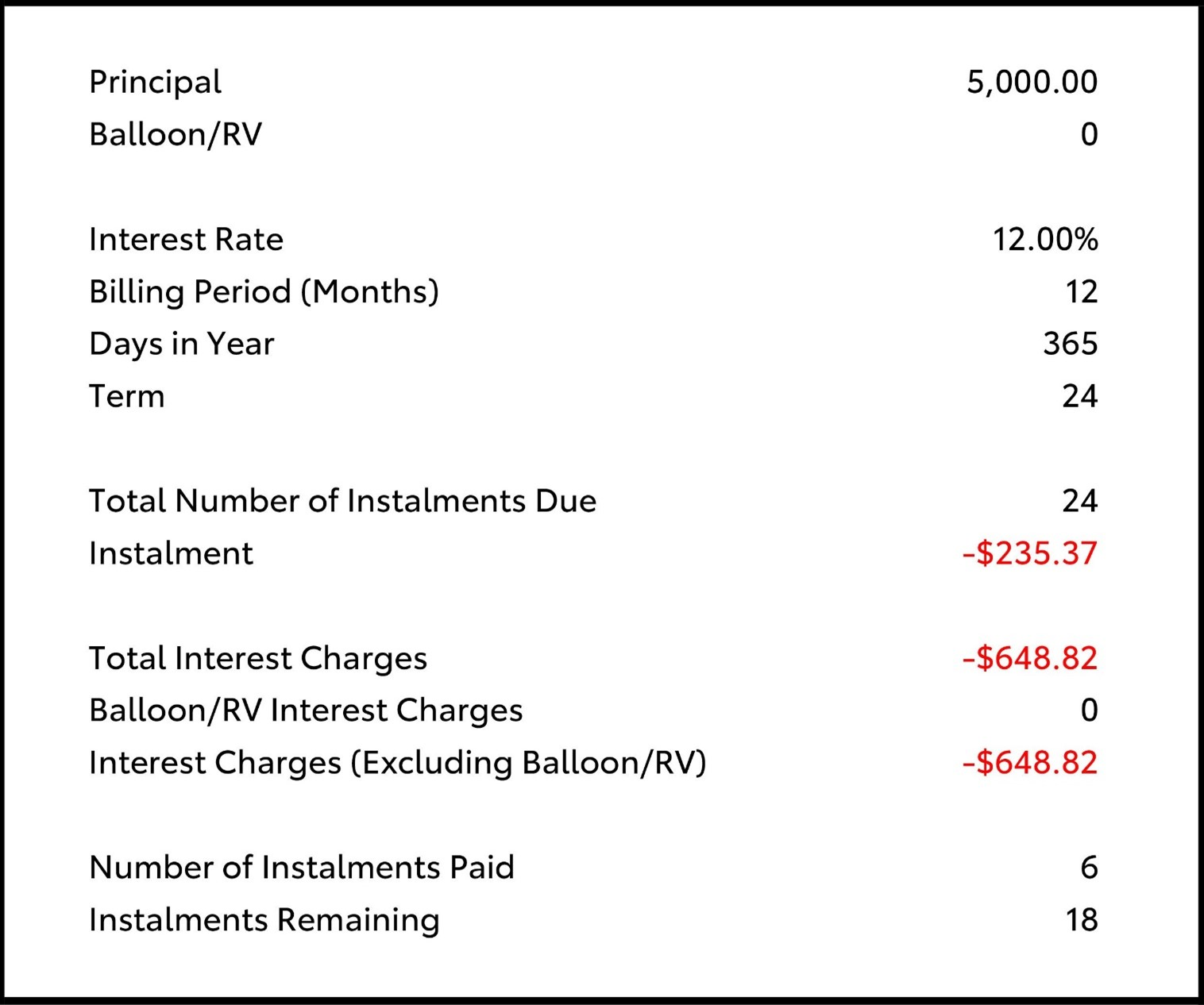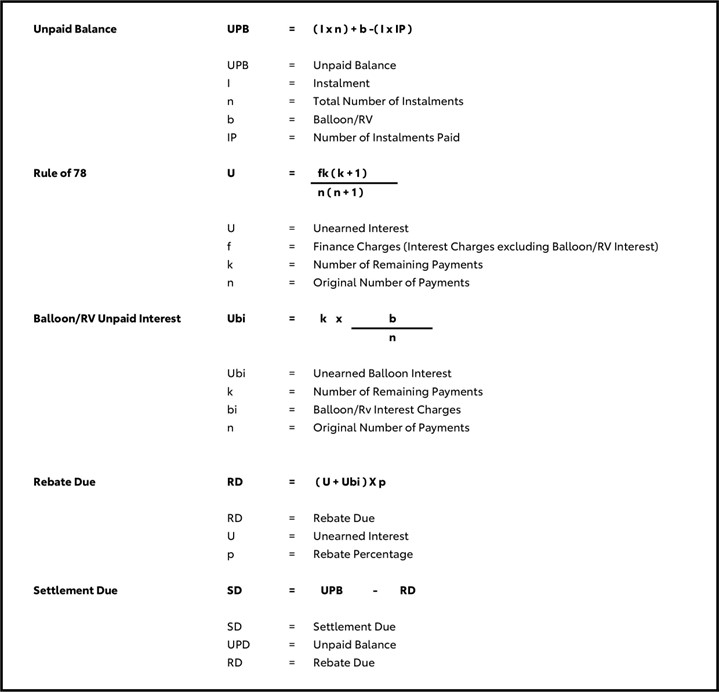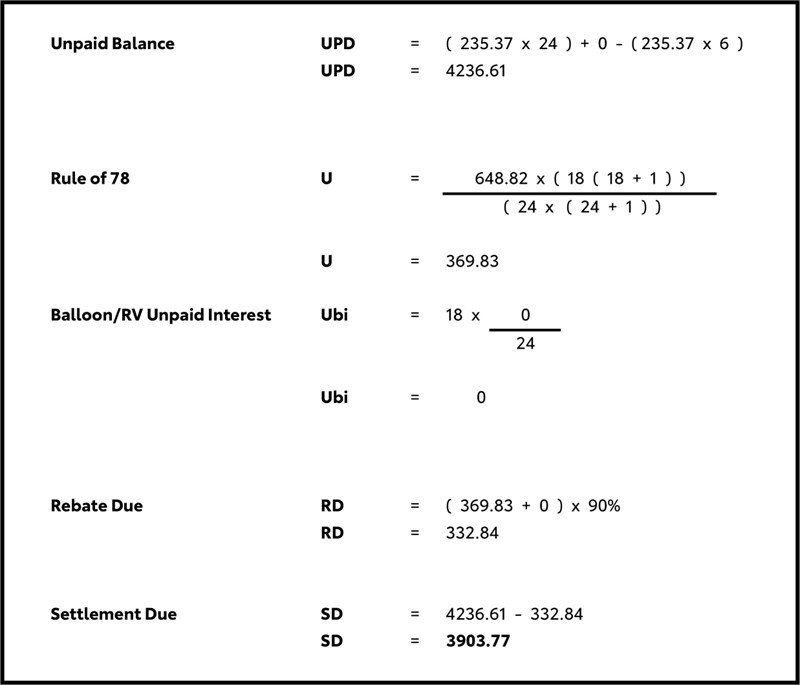## Power Alliance Finance “Rule of 78” Settlement Methodology for Non-Consumer Loans

The “Rule of 78” is a method used by banks and finance companies to break down the principal and interest in the monthly repayment of an instalment loan. Under this rule, the proportion of interest in the monthly payments decreases over the course of the loan period. For example, if a loan is to be repaid over 12 months, the total interest will be divided into 78 portions (12 + 11 + 10 + … + 1 = 78).

12/78ths of the interest is allocated as the first month’s portion of the total interest, 11/78ths of the interest is allocated as the second month’s portion and so on until the twelfth month, at which time 1/78th of the interest is allocated as that month’s portion of the total interest.

The total interest portions for different loan terms are illustrated as below:

Loan Term (Months) Interest Portion
12 + 78 (12 11 + 10 +...+ 1)
24 300 (24 + 23 + 22 +...+ 1)
36 666 (36 + 35 + 34 +...+ 1)
48 1,176 (48 + 47 + 46 +...+ 1)
60 1,830 (60 + 59 + 58 +...+ 1)

When loans are settled early, we use the formula in the example below to ensure we calculate an accurate settlement amount using the “Rule of 78” to calculate the interest component for our loans.

The settlement due in the example below is based on a \$5000, 24-month loan, with 24 regular payments, a 12% p.a. interest rate, that is settled after making 6 regular payments.

## Rule of 78 Settlement Methodology

### Example### Formulas### Example Using Formulas